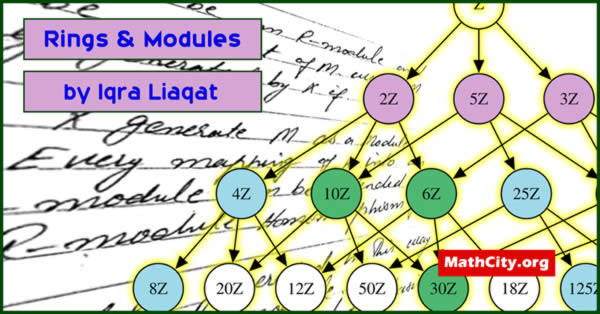# Rings & Modules by Ms. Iqra LiaqatThese notes are send by Ms. Iqra Liaqat. We are really very thankful to her for providing these notes and appreciates her effort to publish these notes on MathCity.org

 Name Rings & Modules Ms. Iqra Liaqat 140 pages PDF (see Software section for PDF Reader) 5.88 mB
• Groupoid
• Semi group
• Group
• Subgroup
• Ring
• Field
• Vector space
• Module
• Unital R module
• Module homomorphism
• Monomorphism
• Epimorphism
• Isomorphism
• Kernal of homomorphism
• Range of T
• Ring homomorphism
• Quotient Module
• First theorem of homomorphism
• Second isomorphism theorem
• Fundamental theorem of homomorphism
• Internal direct sum
• External direct sum
• Finitely generated module
• Cyclic module
• Torsion free module
• Torsion element
• Freely generated module
• Linearly independent & dependent set
• Polynomial ring
• Degree of polynomial
• Constant polynomial
• Remainder theorem
• Zeros of the polynomial
• Roots of the equation
• Factor theorem
• Existence theorem
• Gaussian integer and ring of Gaussian integer
• Prime
• Irreducible element
• Irreducible polynomial over field
• Monic polynomial
• Unique factorization domain (U.F.O)
• Principle ideal
• Principle ideal domain
• Euclidean domain
• Factorization domain

• msc/notes/rings-and-modules-iqra-liaqat